# Polar and Cartesian Coordinates

... and how to convert between them.

To pinpoint where we are on a map or graph there are two main systems:

## Cartesian Coordinates

Using Cartesian Coordinates we mark a point by how far along and how far up it is:## Polar Coordinates

Using Polar Coordinates we mark a point by how far away, and what angle it is:## Converting

To convert from one to the other we will use this triangle: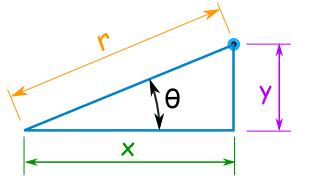## To Convert from Cartesian to Polar

When we know a point in Cartesian Coordinates (x,y) and we want it in Polar Coordinates (r,θ) we solve a right triangle with two known sides.

### Example: What is (12,5) in Polar Coordinates?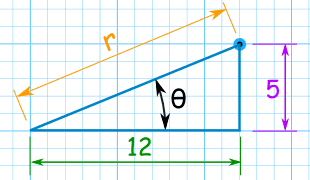Use Pythagoras Theorem to find the long side (the hypotenuse):

r2 = 122 + 52
r = √ (122 + 52)
r = √ (144 + 25)
r = √ (169) = 13

Use the Tangent Function to find the angle:

tan( θ ) = 5 / 12
θ = tan-1 ( 5 / 12 ) = 22.6° (to one decimal)

Answer: the point (12,5) is (13, 22.6°) in Polar Coordinates.### What is tan-1 ?

It is the Inverse Tangent Function:

• Tangent takes an angle and gives us a ratio,
• Inverse Tangent takes a ratio (like "5/12") and gives us an angle.

### Summary: to convert from Cartesian Coordinates (x,y) to Polar Coordinates (r,θ):

• r = √ ( x2 + y2 )
• θ = tan-1 ( y / x )

Note: Calculators may give the wrong value of tan-1 () when x or y are negative ... see below for more.

## To Convert from Polar to Cartesian

When we know a point in Polar Coordinates (r, θ), and we want it in Cartesian Coordinates (x,y) we solve a right triangle with a known long side and angle:

### Example: What is (13, 22.6°) in Cartesian Coordinates?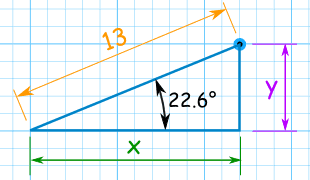Use the Cosine Function for x: cos( 22.6° ) = x / 13 Rearranging and solving: x = 13 × cos( 22.6° ) x = 13 × 0.923 x = 12.002... Use the Sine Function for y: sin( 22.6° ) = y / 13 Rearranging and solving: y = 13 × sin( 22.6° ) y = 13 × 0.391 y = 4.996...

Answer: the point (13, 22.6°) is almost exactly (12, 5) in Cartesian Coordinates.

### Summary: to convert from Polar Coordinates (r,θ) to Cartesian Coordinates (x,y) :

• x = r × cos( θ )
• y = r × sin( θ )

### How to Remember?

(x,y) is alphabetical,
(cos,sin) is also alphabetical

Also "y and sine rhyme" (try saying it!)

## But What About Negative Values of X and Y?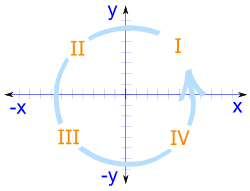When we include negative values, the x and y axes divide the
space up into 4 pieces:

Quadrants I, II, III and IV

(They are numbered in a counter-clockwise direction)

When converting from Polar to Cartesian coordinates it all works out nicely:

### Example: What is (12, 195°) in Cartesian coordinates?

r = 12 and θ = 195°

• x = 12 × cos(195°)
x = 12 × −0.9659...
x = −11.59 to 2 decimal places
• y = 12 × sin(195°)
y = 12 × −0.2588...
y = −3.11 to 2 decimal places

So the point is at (−11.59, −3.11), which is in Quadrant III

But when converting from Cartesian to Polar coordinates ...

... the calculator can give the wrong value of tan-1

It all depends what Quadrant the point is in! Use this to fix things:

 Quadrant Value of tan-1 I Use the calculator value II Add 180° to the calculator value III Add 180° to the calculator value IV Add 360° to the calculator value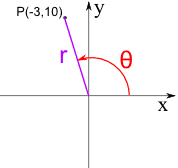### Example: P = (−3, 10)

• r = √((−3)2 + 102)
r = √109 = 10.4 to 1 decimal place
• θ = tan-1(10/−3)
θ = tan-1(−3.33...)

The calculator value for tan-1(−3.33...) is −73.3°

The rule for Quadrant II is: Add 180° to the calculator value
θ = −73.3° + 180° = 106.7°

So the Polar Coordinates for the point (−3, 10) are (10.4, 106.7°)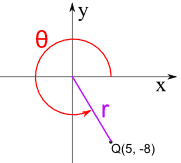### Example: Q = (5, −8)

• r = √(52 + (−8)2)
r = √89 = 9.4 to 1 decimal place
• θ = tan-1(−8/5)
θ = tan-1(−1.6)

The calculator value for tan-1(−1.6) is −58.0°

The rule for Quadrant IV is: Add 360° to the calculator value
θ = −58.0° + 360° = 302.0°

So the Polar Coordinates for the point (5, −8) are (9.4, 302.0°)

## Summary

To convert from Polar Coordinates (r,θ) to Cartesian Coordinates (x,y) :

• x = r × cos( θ )
• y = r × sin( θ )

To convert from Cartesian Coordinates (x,y) to Polar Coordinates (r,θ):

• r = √ ( x2 + y2 )
• θ = tan-1 ( y / x )

The value of tan-1( y/x ) may need to be adjusted:

• Quadrant I: Use the calculator value